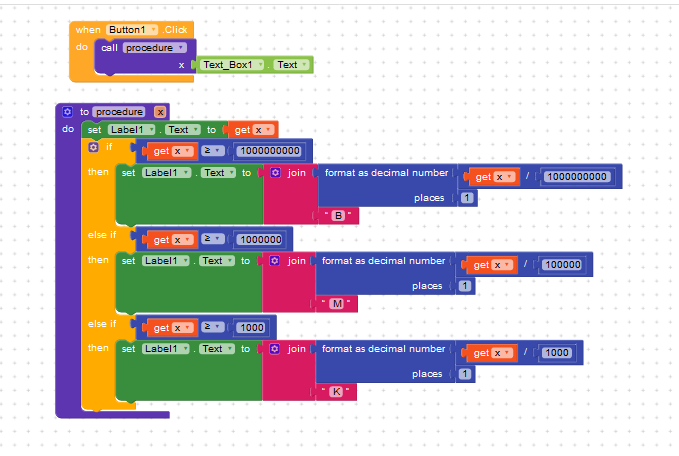# Convert number to Shortcut (1000=1K)

How to change number to Shortcut (1000=1K)
Like 1435=1.4K
Examples
1K
1.1K
547K
(K,M,B)
Use Cases: Views, Likes etc.

Is there any extension for this?
How to do this?

1 Like

i didnt seen any extension for this, maybe if exists anyone knows so they will answer,

mean while you can try to make method of you own, on github i have read that, check this link

How to implement this on Kodular?

by using if, else and else if,
and
“<” this is available in math blocks

@krish.jha if you are free then this is a good idea to make extension.3 Likes

If you want to make extension
if it is a single number
I want 1.1K Format (with 1 decimal place)
else 765K format

convert.aia (2.9 KB)

Check This

2 Likes

We need an extension to make 3 ifs and 3 divisions? Really?2 Likes

We want logic not extension for every thing.
Extension is only for adding some extra features and libraries

2 Likes

I would suggest that you make an algorithm that relies on the log function (actually, if the value could be negative, a log of the absolue value, after ruling out the risk one would be zero).
Then, you divide by 3 to get the multiplier symbol index from a list
large_suffix = {k, M, G, T, P, E Z, Y}

(that is using the prefix defined in SI)
If you want to go on the smaller side as well, the 1/1000, 1/1000000 etc. symbols are
small_suffix = {m, μ, n, p, f, a, z, y}

The log of this is 4.75128.
If you divide by 3, you have 1.5837, which you can round down (floor) as 1. Therefore you can divide your value 56400.3 by 10^(1*3) and get 56.4003, then tag the symbol “k”, since this is large_suffix.

For a value in the million, the result of the log / divide / round_down_floor process is 2, therefore you divide the value by 10^(2*3) [one million) and append the second suffix form the list, which would be M.

1 Like

@pavi2410 wondering if this can be included to Kodular Utility Blocks1 Like

I have an extension to that can do that but not finding the thread where I downloaded that

Extension is
com.avm.ViewsConvert.aix

4 Likes